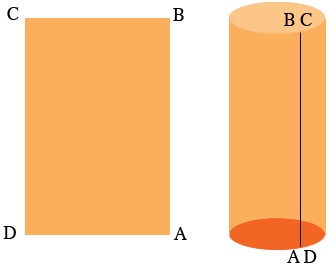SEARCH HOMEMath Central Quandaries & QueriesQuestion from Amanda: How do I find the maximum surface area and volume of a cylinder made up of ONE 8.5x11 piece of paper?Hi Amanda,

The surface area part of this question is easy. If you can use the entire sheet of paper then the surface area is $8.5 \times 11$ square inches and you can't obtain a larger surface area.

How do you construct a cylinder using the entire sheet of Paper? On way is to place the sheet with the 8.5 inch side in the horizontal direction and the 11 inch side in the vertical direction.Roll up the sheet so that the edges labeled AB and CD line up and tape them together as in the diagram. You now have a cylinder. The volume of a cylinder is $\pi r^ h$ where $r$ is the radius of the base and $h$ is the height. The height is the distance from $A$ to $B$ and the circumference of the base is the distance from $A$ to $D.$ What is the equation for the circumference of a circle? If the circumference is 8.5 inches what is its radius? What is the volume of the cylinder?

Now roll the sheet in the opposite direction al tape the sides BC and AD together. What is the volume of this cylinder?

Which is larger?

Take two sheets of 8.5 by 11 paper and form the two cylinders. Which one looks like it has a larger volume? Does you visual intuition agree with your calculations?

PennyMath Central is supported by the University of Regina and The Pacific Institute for the Mathematical Sciences.